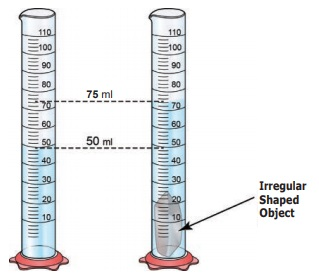Home | | Science 6th Std | Volume

# Volume

Length is a fundamental quantity. That means, it connot be expressed in any other quantities. Using length, we can find out other measurements like Area and Volume. Area is obtained by using two lengths. So, Area = Length × Breadth.

Volume

1. Volume of Solids

Length is a fundamental quantity. That means, it connot be expressed in any other quantities. Using length, we can find out other measurements like Area and Volume. Area is obtained by using two lengths. So, Area = Length × Breadth. Now, you can guess how to measure the area of your text book, your classroom or your playground.Volume is also a derived quantity and it can be measured from measuring lengths. Let us calculate volume of a box. To know the volume of a box we need to know the length (l), breadth (b) and height (h). With a measuring scale measure the three parameters in cm. The volume of the box = l × b × h. Unit of volume would be obviously cm × cm × cm = cubic cm or cm3. What do you infer from this? Assume the volume of cubical box is 1000 cubic cm. It means 1000 cubes each with dimensions 1cm × 1cm can be placed inside the box. Try to express this quanity in suitable unit.

2. Volume of Liquid

Measuring the volume of a liquid is quite easy. Liquids take the shape of the container in which they are kept. A liquid whose volume is to be found can simply be poured into a graduated container. Graduated cylinders, beakers, pipettes and burettes are available for measuring exact volumes. The volume of liquid is usually measured in litres.We would have often heard the word litre in our daily life. For an example 1 litre milk packet, 20 litres water can.

Do you know the quantity of blood in a human body?

Measuring the volume of objects with irregular shape

Suppose you want to measure the volume of an object which has irregular shape. How will you do this? There is no mathematical formula to measure this but there are many ways to do. Remember, volume is the space occupied by an object. We can use this property to measure the volume of an object with irregular shape, for example a stone.

Let us try to find volume of a small piece of stone.Fill a graduated measuring cylinder with water to certain level, say 50 ml. Tie the stone with a piece of fine thread. Immerse the stone completely into water.

As the stone is immersed, we can observe that water level increases. Why? The stone displaces the water to occupy the space inside the measuring cylinder. How much water would be displaced? It would be equal to the space taken up by stone. So the amount of water displaced will be the volume of the stone. This method is called as water displacement method.

Suppose initially the water level was 50 ml. After you immerse a stone the water level rises to 75 ml. What is the volume water displaced? Volume of the water displaced is 75 ml – 50 ml = 25ml

1ml = 1 cubic cm

25ml  =  25 cm3. the volume of stone = 25cm3

3. Volume of gas

We can also measure the volume of gases. Gases expand to fill the container into which they are placed. When you compress, a gas you can make the same gas to occupy lesser space. (as in LPG gas cylinder). Therefore it is not easy to talk about the volume of gas in the same way as a volume of a stone or a liquid. It is essential to take into account the pressure at which the gases are kept. We will learn about these later.

SI unit for Volume of solid is cubic metre. Liquids and gases are usually measured in litres. But also can be measured in m3 or cubic metre. Similar to the length,

1 m3 = 1 kilolitre (kl or kL)

1cm3 = 1 millilitre (ml or mL)

1mm3 = 1 microlitre (µl or µL)

Activity 4

Take three vessels filled with water. By looking at the amount of water guess which vessel has more volume of water. How will you check this experimentally?

How to measure the volume of the given vessel?By looking at the amount of water we guess that cylinder vessel has more volume of water.

Experimentally we measure that water by liter beaker.

To measure the volume of the given vessel by kilo litre.

Tags : Measurements | Term 1 Unit 1 | 6th Science , 6th Science : Term 1 Unit 1 : Measurements
Study Material, Lecturing Notes, Assignment, Reference, Wiki description explanation, brief detail
6th Science : Term 1 Unit 1 : Measurements : Volume | Measurements | Term 1 Unit 1 | 6th Science